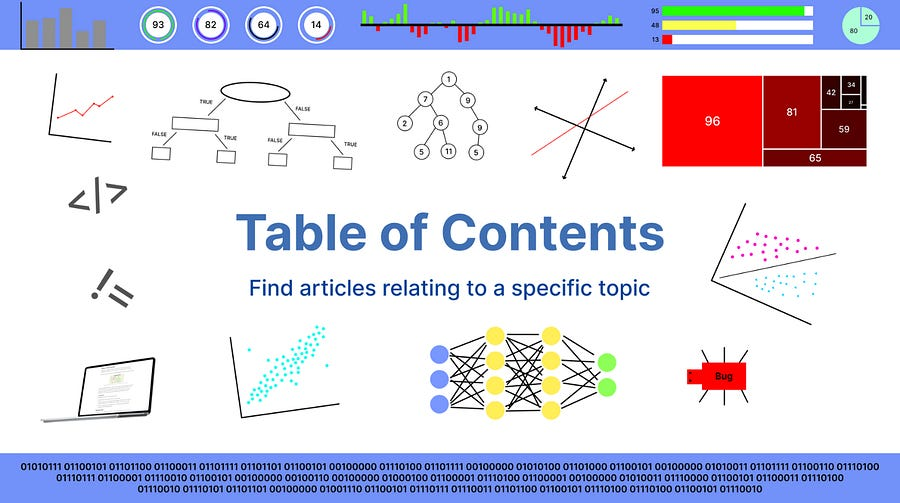Here you can find articles relating to a specific topic you’re looking for. A way to keep things organized for you.
This is the fourth introduction to R. This will cover functions, while loops, for loops, built-in features, and more.
This is the third introduction to R. This will cover the basics of data frame, data frame indexing and selection, operations, and more.
This is the second introduction to R. This will cover matrix, matrix operations, factor matrices, and more.
The first introduction to R for data science. This will cover arithmetic, variables, vector basics, and more.
A guide on how to improve software applications.
The second introduction to Data Science. This will cover k-nearest neighbors, support vector machines, neural networks, and more.
An introduction to Data Science. This will cover the basics of data, the selection of data, results, and more. Note that this does not contain any math…
See all